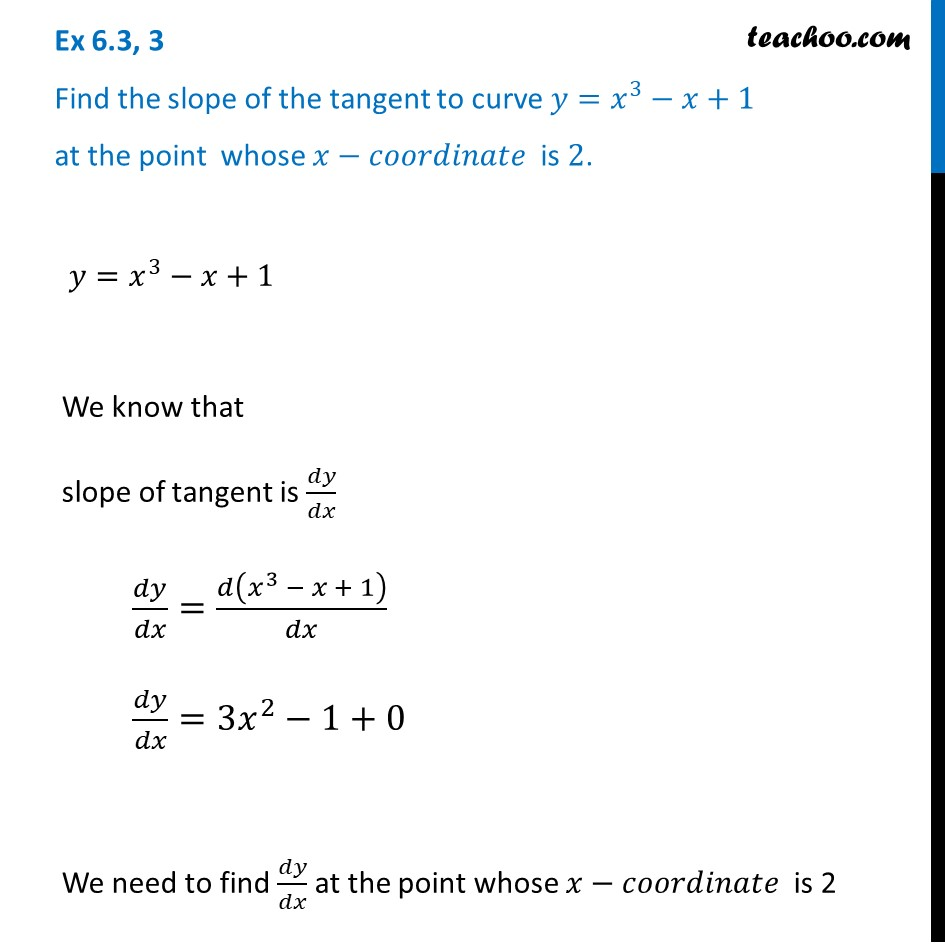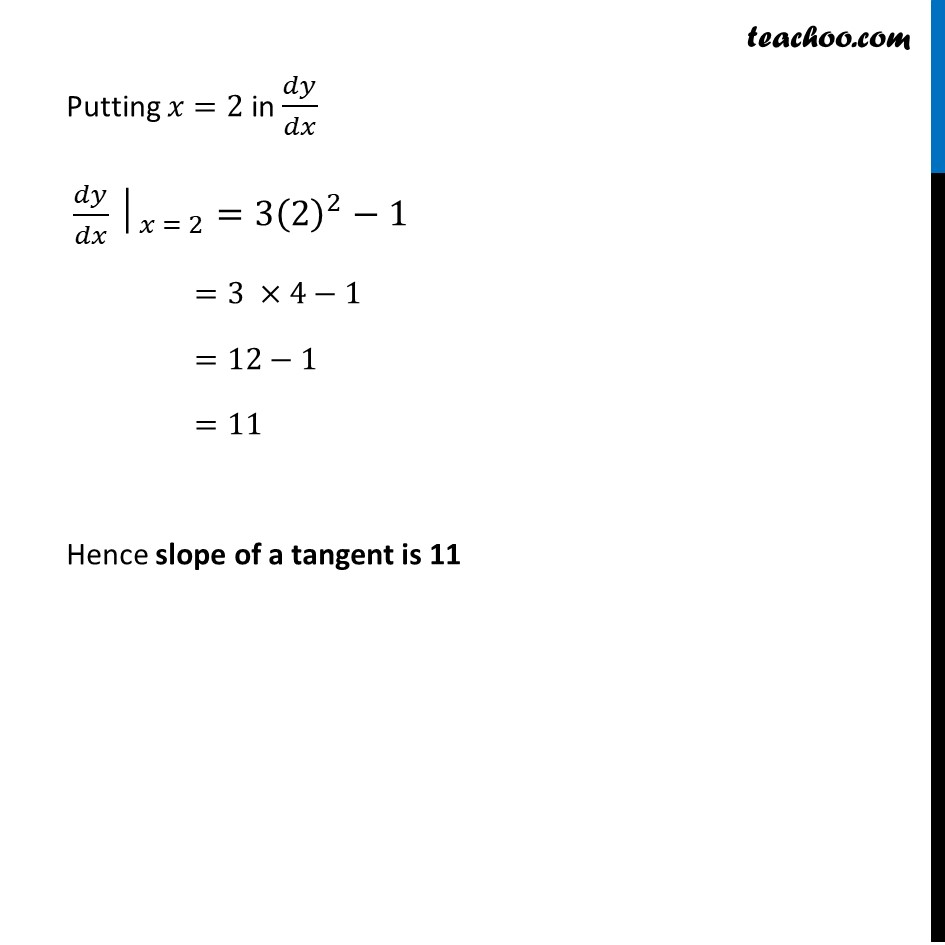Tangents and Normals (using Differentiation)

Chapter 6 Class 12 Application of Derivatives
Serial order wiseLearn in your speed, with individual attention - Teachoo Maths 1-on-1 Class

### Transcript

Question 3 Find the slope of the tangent to curve 𝑦=𝑥^3−𝑥+1 at the point whose 𝑥−𝑐𝑜𝑜𝑟𝑑𝑖𝑛𝑎𝑡𝑒 is 2. 𝑦=𝑥^3−𝑥+1 We know that slope of tangent is 𝑑𝑦/𝑑𝑥 𝑑𝑦/𝑑𝑥=𝑑(𝑥^3 − 𝑥 + 1)/𝑑𝑥 𝑑𝑦/𝑑𝑥=3𝑥^2−1+0 We need to find 𝑑𝑦/𝑑𝑥 at the point whose 𝑥−𝑐𝑜𝑜𝑟𝑑𝑖𝑛𝑎𝑡𝑒 is 2 Putting 𝑥=2 in 𝑑𝑦/𝑑𝑥 〖𝑑𝑦/𝑑𝑥│〗_(𝑥 = 2)=3(2)^2−1 =3 ×4−1 =12−1 =11 Hence slope of a tangent is 11